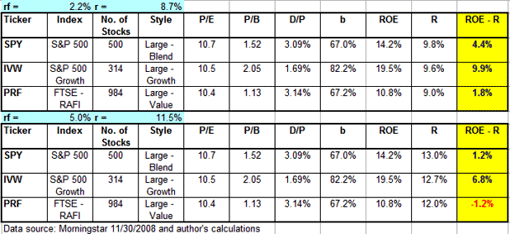# The Hidden Value

January 02, 2009

Examining the 'margins of safety' between expected return and actual return on equity for index funds.

Towards the end of the last century, academic finance economists came to take seriously the view that aggregate stock returns are predictable."

This somewhat surprising statement comes from John Y. Campbell an economics professor at Harvard University and Samuel B. Thompson, manager of research in Arrowstreet Capital, L.P., in an article that they published recently.

Campbell and Thompson investigated whether value ratios, such as P/E, P/B and D/P, were helpful in predicting the future returns of the overall stock market during the period of 1926-2005. Their first conclusion was that value ratios showed an ability to predict future returns, e.g., periods with low P/E or conversely high D/P ratios were usually followed by periods with higher-than-normal returns in the stock market.

This conclusion should not come as a surprise to value investors, who follow the footsteps of Benjamin Graham, but it may raise some eyebrows in the academy. The second, and more interesting conclusion, was that the best predictions of future returns were obtained once the parameters of the forecast model were computed using the Dividend Growth Model, known also as the Gordon Model.

The Gordon Model, in its simplest form, determines the relation between the dividend yield (D/P), the expected growth in dividends (g) and the expected rate of return (r).

(1)     D/P = r - g

In a previous article ("The Risk Premium Puzzle"), I showed that this simple relation can be used to detect abnormally large or small risk premiums. Another extension of the Gordon Model is the Abnormal Earnings Model (AEM). The AEM, which is also known as the Residual Income Model, can be derived from the Gordon Model with the addition of the long-term relation between the dividend growth rate (g), the return on equity (R) and the earnings retention rate (b):

(2)     g = R * b

The retention rate (b) is the percentage of earnings (E) that is not paid out as dividends (D), but rather, is retained to support future growth:

(3)     b = 1 - D/E = 1 - (D/P) * (P/E)

Using equations (1) and (2), the derivation of the AEM is fairly straightforward (for the interested reader, I have added an appendix that presents the mathematical proof of (2) and (4) and some other methodological issues that are related to the calculations hereinafter):

(4)     P/B  =  1 + (R - r) / (r - R*b)

Campbell and Thompson used equations (1) and (4) to predict the expected rate of return on the stock market. I propose a slightly different approach: From equation (4), it is possible to extract the expected return on equity (R):

(5)     R = (P/B) * r / (1 + b * ((P/B) - 1))

The expected return on equity (R) is the level of profitability that conforms to the current level of price and the expected rate of return. However, it is not necessarily equal to the actual return on equity (hereinafter ROE) or to the historical level of the return on equity. Comparing the level of R to either the actual ROE or to the historical level of the return on equity can provide a useful insight on the current level of prices. High or low levels of R, in comparison to these benchmarks, may serve as a sign that stocks are over- or undervalued.

Based on data from Morningstar, I performed such comparison for three ETFs that follow stock indexes of large American corporations: SPY—which follows the S&P 500; IVW—which follows the S&P 500 Growth Index; and PRF—which is a fundamental index that follows the RAFI 1000, the fundamental equivalent of a large-cap U.S. index. These indexes represent the three investment styles defined by Morningstar: Large Blend (SPY), Large Growth (IVW) and Large Value (PRF).

The results of the comparison are presented in Table 1.

Table 1